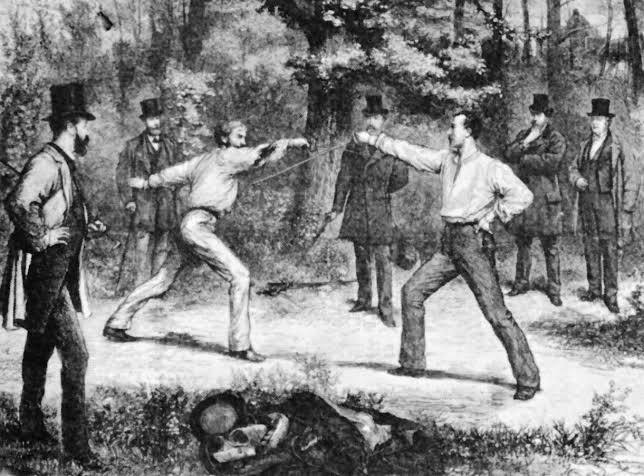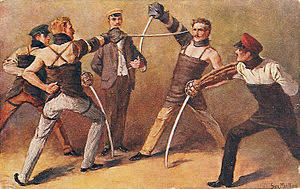# Study Challenge

Hello beautiful minds! This challenge is meant for everyone who is dedicated to study consistently and make their future great.
I welcome you with the old school motto

Read the 3rd post of this thread for the details.
If you wish to join, add your name to the 6th post of this thread.

22 Likes

Hoi… I really want to join this challenge… thanks for starting it again…# Details and rules

• This is going to be an individual challenge, each member is a lone warrior.

• Each warrior is required to note their study time everyday either manually or by using some timer app. Those who are having classes add your class time too, but only add productive time out of them, like if you are having breaks in between classes don’t add that time.

• The scoring system is
0.5 points for half an hour study, 1 point for one hour study and so on. The score should be rounded to lower number i. e. 4 hrs and 50 min counts as 4.5 points and not 5

• Each warrior is required to post their score in the scoreboard as often as possible, posting study time in the thread daily is preferable, posting screenshot of your study hours is optional.

The main challenge will run for an entire month and each month names of top three scorers will be added to the hall of fame and the person whose score is highest at the end of the month will be the King of warriors for that particular month

Along with the main challenge there will be special events

Once someone joins this challenge they will be considered an all time member and their names will automatically be added to the scoreboard each month, if someone wants to leave or take a break at anytime just tag @Samaranjay

Failing to update the score by 2nd date of the upcoming month will lead to elimination (you’ll be reminded around the end of the month about it)

# Special events

## DuelsIn the first 5 days of the month any two warriors can challenge each other, one duel per day, the one who wins the duel at the end of the day 5 points will be added to their month’s counter.

## Group battleThis event will occur twice a month for three days each from 15th-17th and then from 25th-27th, only brave warriors who have sheer determination can join these.
Instructions for the members who join the battle :

1. Completing 6 hours or more on each day will get +5 points for the month.
2. Failing to complete 18 hours of study in these 3 days will lead to reduction of 5 points from your monthly score i.e -5 points
3. If you fail to complete 6 hours on a particular day out of 3 days and still manage to complete 18 hours in 3 days then no points will be added or subtracted from your monthly tally.
4. The warrior who studies the most in these 3 days will get +15 points.
9 Likes

# Hall of fame

Month 1st (King of warriors) 2nd 3rd
May TheBigSP shiva25 sheamus
June

## Runners up section

Month 4th 5th 6th
May Samaranjay _TIGER raushan
June
3 LikesBro and what about those chambers?? As Ash Matt did?

2 Likes

# Scoreboard

New Participants

Participants who are already in the challenge.

1. TheBigSP Duel points 20
(6.5) +(7.5) +(9) +(9) +(8) +(7.5) +(8.5) +(7) +(7) +() +() +() +() +() + () +() +() +() +() +() +() +() +() +() +() = ()
1. shiva25 Duel points 5
(5.5) +(11) +(6) +(9) +(9) +() +() +() +() +() +() +() +() +() + () +() +() +() +() +() +() +() +() +() +() = ()
1. Sheamus Duel points 5
(5.5) +(7.5) +(8) +(3.5) +(7.5) +(6.5) +(4) +() +() +() +() +() +() +() + () +() +() +() +() +() +() +() +() +() +() = ()
1. Samaranjay Duel points 10
(4) +(2.5) +(2.5) +(0) +(0) +(1) +(0) +(1.5) +(6) +() +() +() +() +() + () +() +() +() +() +() +() +() +() +() +() = ()
1. _TIGER Duel points 15
() +() +() +() +() +() +() +() +() +() +() +() +() +() + () +() +() +() +() +() +() +() +() +() +() = ()

2. SincereDev
(6) +(6) +(1) +(1) +(6) +(3.5) +(2.5) +(3.5) +() +() +() +() +() +() + () +() +() +() +() +() +() +() +() +() +() = ()

3. maya Duel points 5
(8) +(7) +(5) +() +() +() +() +() +() +() +() +() +() +() + () +() +() +() +() +() +() +() +() +() +() = ()

4. Karan050
(6) +(5.5) +(3) +(0) +(7) +(3) +(5.5) +(0) +() +() +() +() +() +() + () +() +() +() +() +() +() +() +() +() +() = ()

5. Prox
() +() +() +() +() +() +() +() +() +() +() +() +() +() + () +() +() +() +() +() +() +() +() +() +() = ()

6. The monk
(5) +(6) +(5) +(5) +(2.5) +(6) +(1) +(0.5) +(1.5) +() +() +() +() +() + () +() +() +() +() +() +() +() +() +() +() = ()

7. Dean Duel points 10
(0) +(0) +(1) +(4) +(6) +() +() +() +() +() +() +() +() +() + () +() +() +() +() +() +() +() +() +() +() = ()

1. Kanu Duel points 15
() +() +() +() +() +() +() +() +() +() +() +() +() +() + () +() +() +() +() +() +() +() +() +() +() = ()
1. rewire_user
() +() +() +() +() +() +() +() +() +() +() +() +() +() + () +() +() +() +() +() +() +() +() +() +() = ()

2. ysub
() +() +() +() +() +() +() +() +() +() +() +() +() +() + () +() +() +() +() +() +() +() +() +() +() = ()

3. drago
() +() +() +() +() +() +() +() +() +() +() +() +() +() + () +() +() +() +() +() +() +() +() +() +() = ()

4. MAHESH27_03 Duel points 5
() +() +() +() +() +() +() +() +() +() +() +() +() +() + () +() +() +() +() +() +() +() +() +() +() = ()

5. RaZat
(4.5) +(7) +(7) +(2.5) +(2.5) +(2) +(7) +(7) +(7) +() +() +() +() +() + () +() +() +() +() +() +() +() +() +() +() = (46.5)

6. NhTbH
(4.5) +(0) +(7.5) +(0) +(0) +(0) +(3) +(1) +() +() +() +() +() +() + () +() +() +() +() +() +() +() +() +() +() = ()

1. neo_150
(2) +(1) +(4.5) +(2.5) +(5.5) +(2) +() +() +() +() +() +() +() +() + () +() +() +() +() +() +() +() +() +() +() = ()
1. Mugiwara41
(2) +() +(2) +() +(2) +() +() +(2) +() +(2) +() +(2) +() +() + () +() +() +() +() +() +() +() +() +() +() = ()

2. Evolved
() +() +() +() +() +() +() +() +() +() +() +() +() +() + () +() +() +() +() +() +() +() +() +() +() = ()

3. Bruhdude
() +() +() +() +() +() +() +() +() +() +() +() +() +() + () +() +() +() +() +() +() +() +() +() +() = ()

# Scoreboard

## Group battle log

Month 1st battle participants Winner 2nd battle participants Winner
May Samaranjay TheBigSP
June 4,12, 2,1,19,3
13 LikesI think this one is better… All the best bro… see you tomorrow… Have a nice night… Good Night

3 Likes

@_TIGER @Dean_Ambrose @Guichota @rohankians @SincereDev @sheamus @shiva25 @prox @Ash_Matt @Mahesh27_03
Guys read the details of the challenge and you are most welcome to join if you find this Challenge worthwhile

7 Likes

# As you all might have noticed that it is already 7th May so there will be no duels this month, we’ll be waiting for new members to join until 9th May and will begin the main challenge from 10th May, the group wars will commence as prescribed.

Those who have already joined can take 8th and 9th May as practice days for the main challenge

8 Likes

Thanks for the ping I’m inSo are we supposed to send ss from some productivity app or just send the time?

Edit : ok I’ve read the rules now dumb me2 Likesno problem bro, it happens.

1 Like

Count me in brother.
I will give my best.3 Likes

Cool! Added you name to the scoreboard @rewire_user

1 Like

@Samaranjay you told us about extreme productive thread and now you made new study thread?4 Likes

It was my responsibility to maintain the study challenge bro, that’s why I created this thread as soon as I realised that I was just finding an excuse to not handle this responsibility.

6 Likes

I am also in guys… Let’s make our days productive

2 Likes

8th may (practice day)

1 hour 55 minutes of focus today (slept most of the day)

2 Likes

Im in… Ready to ramp up my study hours

3 Likes

That’s good, do better tomorrow, keep improving., You are already doing great bro, just maintain it consistently.

5hrs 40 min study today. Not enough, have to improve a lot to get into the hall of fame as I know what potential current participants have.
Let’s Rock the month3 Likes

@Samaranjay hey buddy add me in the challenge !!

3 Likes